# Magnetic dipole moment of a current-carrying loop, derivation class 12

• 1
•
• 1
•
•
•
•
•
2
Shares

In this section, we will discuss and derive the expression for the magnetic dipole moment of a circular current-carrying loop, so let’s get started…

## Magnetic dipole

It is the system of two equal and opposite magnetic poles separated by a small distance called a magnetic dipole.

## Magnetic dipole moment

The magnetic dipole moment of a magnetic dipole is the product of the pole strength and the distance between their poles i.e magnetic length. It is a vector quantity and is always directed from the South pole to the North pole. Units of magnetic dipole moment are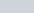## Magnetic dipole moment of a circular current carrying loop, derivationFig. 1, Magnetic dipole moment of a circular current-carrying loop, source: toppr.com

If you observed a circular current carrying loop then you will find that it behaves likes a magnetic dipole. Why am I saying this? If you look at the upper face, then you will find that the direction of the current is anticlockwise, so it has north polarity. But if you look at the lower face, then you will find that the current is clockwise, so it has south polarity or south pole.

That means the current loop behaves like a system of two equal and opposite magnetic poles, hence a circular current carrying loop is an example of a magnetic dipole.

The magnetic dipole moment of a circular current carrying loop is given as-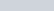where,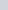is the current flowing through the current loop, and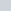is the area enclosed by the circular loop, and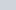is the magnetic dipole moment.

The magnitude of the magnetic field due to a circular current-carrying loop of steady current I and having radius R at any point on its axis at distance x is given by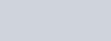If x>>>R, then the above expression can be rewritten as-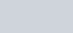Multiply numerator and denominator by π, we get-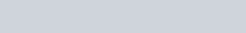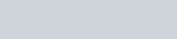If the current loop has N turns, then the magnetic dipole moment is –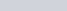The magnetic dipole moment of a circular current carrying loop is defined as the product of the current flowing and area enclosed. Its direction is perpendicular to the plane of the loop and can be given by the right-hand thumb rule. Watch this video for more understanding.

Stay tuned with Laws Of Nature for more useful and interesting content.